#### Spreading waves

Figure 4.1 shows how waves would propagate from a hypothetical point light source. The density would be the same in all directions (radial symmetry), but would decrease as the light source becomes more distant. Recall that the surface area of a sphere with radius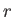is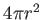. Consider centering a spherical screen around the light source. The total number of photons per second hitting a screen of radius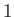should be the same as for a screen of radius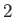; however, the density (photons per second per area) should decrease by a factor of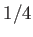because they are distributed over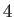times the area. Thus, photon density decreases quadratically as a function of distance from a point light source.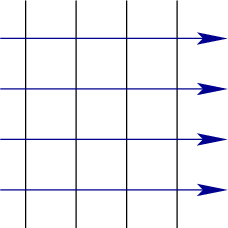The curvature of the wavefronts also decreases as the point light source becomes further away. If the waves were to propagate infinitely far away, then they would completely flatten as shown in Figure 4.2. This results in the important case of parallel wavefronts. Without the help of lenses or mirrors, it is impossible to actually obtain this case from a tiny light source in the physical world because it cannot be so far away; however, it serves as both a useful approximation for distant light sources and as an ideal way to describe lenses mathematically. Keep in mind that at any finite distance from a point light source, the rays of light always diverge; it is impossible to make them converge without the help of lenses or mirrors.

Steven M LaValle 2020-11-11